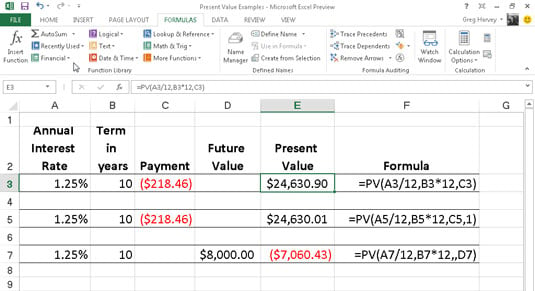##### Excel 2013 All-in-One For DummiesThe PV (Present Value) function in Excel 2013 is found on the Financial button’s drop-down menu on the Ribbon’s Formulas tab (Alt+MI). The PV function returns the present value of an investment, which is the total amount that a series of future payments is worth presently.

The syntax of the PV function is as follows:

`=PV(rate,nper,pmt,[fv],[type])`

The fv and type arguments are optional arguments in the function (indicated by the square brackets). The fv argument is the future value or cash balance that you want to have after making your last payment. If you omit the fv argument, Excel assumes a future value of zero (0).

The type argument indicates whether the payment is made at the beginning or end of the period: Enter 0 (or omit the type argument) when the payment is made at the end of the period, and use 1 when it is made at the beginning of the period.

The figure contains several examples using the PV function. All three PV functions use the same annual percentage rate of 1.25 percent and term of 10 years. Because payments are made monthly, each function converts these annual figures into monthly ones.

For example, in the PV function in cell E3, the annual interest rate in cell A3 is converted into a monthly rate by dividing by 12 (A3/12). The annual term in cell B3 is converted into equivalent monthly periods by multiplying by 12 (B3 x 12).Note that although the PV functions in cells E3 and E5 use the rate, nper, and pmt (\$218.46) arguments, their results are slightly different.

This is caused by the difference in the type argument in the two functions: the PV function in cell E3 assumes that each payment is made at the end of the period (the type argument is 0 whenever it is omitted), whereas the PV function in cell E5 assumes that each payment is made at the beginning of the period (indicated by a type argument of 1).

When the payment is made at the beginning of the period, the present value of this investment is \$0.89 higher than when the payment is made at the end of the period, reflecting the interest accrued during the last period.

The third example in cell E7 uses the PV function with an fv argument instead of the pmt argument. In this example, the PV function states that you would have to make monthly payments of \$7,060.43 for a 10-year period to realize a cash balance of \$8,000, assuming that the investment returned a constant annual interest rate of 1 1/4 percent.

Note that when you use the PV function with the fv argument instead of the pmt argument, you must still indicate the position of the pmt argument in the function with a comma (thus the two commas in a row in the function) so that Excel doesn’t mistake your fv argument for the pmt argument.